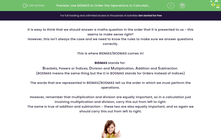# Use BIDMAS to Order the Operations in Calculations

In this worksheet, students will learn how to use the rules of BIDMA/BODMAS correctlyKey stage:  KS 2

Curriculum topic:   Number: Addition, Subtraction, Multiplication and Division

Curriculum subtopic:   Carry Out Calculations Using Order of Operations

Difficulty level:#### Worksheet Overview

It is easy to think that we should answer a maths question in the order that it is presented to us - this seems to make sense right?

However, this isn't always the case and we need to know the rules to make sure we answer questions correctly.

This is where BIDMAS/BODMAS comes in!

BIDMAS stands for:

Brackets, Powers or Indices, Division and Multiplication, Addition and Subtraction

(BODMAS means the same thing but the O in BODMAS stands for Orders instead of Indices)

The words that are represented in BIDMAS/BODMAS tell us the order in which we must perform the operations.

However, remember that multiplication and division are equally important, so in a calculation just involving multiplication and division, carry this out from left to right.

The same is true of addition and subtraction - these two are also equally important, and so again we should carry this out from left to right.Examples

5 + 15 ÷ 5 = 5 + 3 = 8 (divide first)

(12 + 9) ÷ 3 = 21 ÷ 3 = 7 (brackets first)

22 - 3 × 6 = 22 - 18 = 4 (multiply first)

(34 - 4) × 2 = 30 × 2 = 60 (brackets first)

4 × 52 = 4 × 25 = 100 (power first)

(2 × 5)2 = 102 = 100 (brackets first)

34 + 4 - 8 = 30 (equally important, so left to right)

Now, why don't you have a go at answering some questions using BIDMAS.

You can always come back and have another look at this page if you get stuck by clicking on the red help button on the screen.Good luck!

### What is EdPlace?

We're your National Curriculum aligned online education content provider helping each child succeed in English, maths and science from year 1 to GCSE. With an EdPlace account you’ll be able to track and measure progress, helping each child achieve their best. We build confidence and attainment by personalising each child’s learning at a level that suits them.

Get started••••